# Seaborn之绘图风格设置

2019-11-07 11:40    阅读 1284

### Seaborn简介

seaborn同matplotlib一样，也是Python进行数据可视化分析的重要第三方包。但seaborn是在 matplotlib的基础上进行了更高级的API封装，使得作图更加容易，图形更加漂亮。

seaborn并不能替代matplotlib。虽然seaborn可以满足大部分情况下的数据分析需求，但是针对一些特殊情况，还是需要用到matplotlib的。换句话说，matplotlib更加灵活，可定制化，而seaborn像是更高级的封装，使用方便快捷。

## 绘图风格设置

``import pandas as pdimport numpy as npimport matplotlib.pyplot as pltimport matplotlib as mpl``

``def sinplot(flip = 1):    x = np.linspace(0, 14, 100)    for i in range(1, 7):        plt.plot(x, np.sin(x + i * .5) * (7 - i) * flip)sinplot()``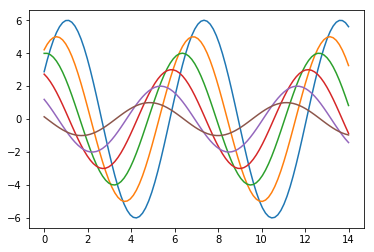seaborn1.png

``import seaborn as snssns.set()sinplot()``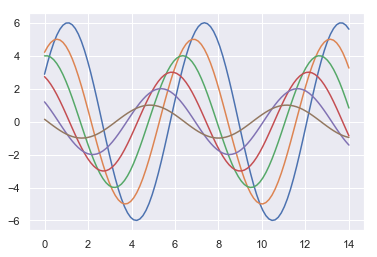seaborn2.png

Seaborn 将 matplotlib 的参数划分为两个独立的组合。第一组是设置绘图的外观风格的，第二组主要将绘图的各种元素按比例缩放的，以至可以嵌入到不同的背景环境中。

### Seaborn的五种绘图风格

``sns.set_style('whitegrid')data = np.random.normal(size = (20, 6)) + np.arange(6) / 2sns.boxplot(data = data)``
``<matplotlib.axes._subplots.AxesSubplot at 0x1a1ae6da20>``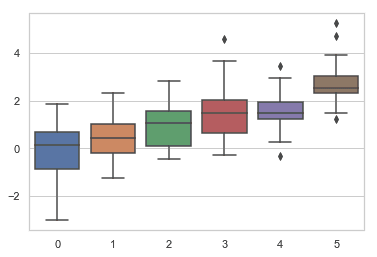seaborn3.png

``sns.set_style('dark')sinplot()``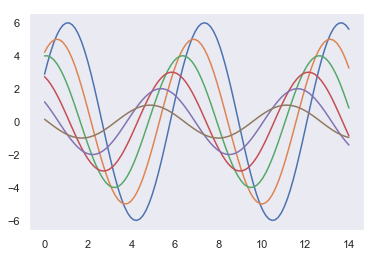seaborn4.png

``sns.set_style('white')sinplot()``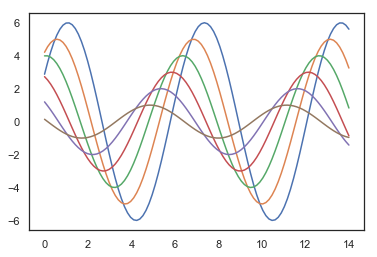seaborn5.png

``sns.set_style('ticks')sinplot()``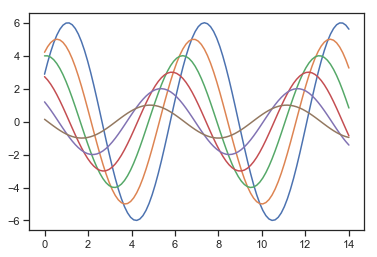seaborn6.png

### 移除轴脊柱

white和ticks两种风格都可以移除顶部和右侧的不必要的轴脊柱。使用matplotlib是无法实现这一需求的，但是使用seaborn的despine()方法可以实现。

``sinplot()sns.despine()``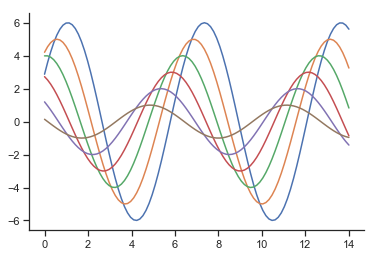seaborn7.png

``f, ax = plt.subplots()sns.violinplot(data=data)sns.despine(offset=10, trim=True)``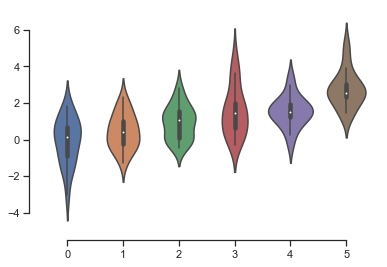seaborn8.png

``sns.set_style("whitegrid")sns.boxplot(data=data, palette="deep")sns.despine(left=True)``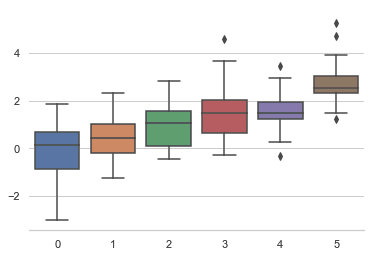seaborn9.png

### 临时设置绘图风格

``with sns.axes_style("darkgrid"):    plt.subplot(211)    sinplot()plt.subplot(212)sinplot(-1)``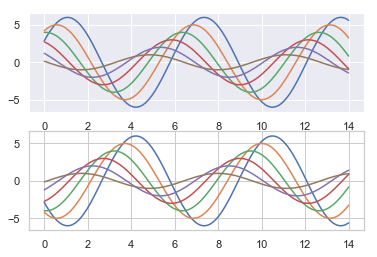seaborn10.png

### 覆盖seaborn风格元素

sns.axes_style()

``{'axes.facecolor': 'white', 'axes.edgecolor': '.8', 'axes.grid': True, 'axes.axisbelow': True, 'axes.labelcolor': '.15', 'figure.facecolor': 'white', 'grid.color': '.8', 'grid.linestyle': '-', 'text.color': '.15', 'xtick.color': '.15', 'ytick.color': '.15', 'xtick.direction': 'out', 'ytick.direction': 'out', 'lines.solid_capstyle': 'round', 'patch.edgecolor': 'w', 'image.cmap': 'rocket', 'font.family': ['sans-serif'], 'font.sans-serif': ['Arial',  'DejaVu Sans',  'Liberation Sans',  'Bitstream Vera Sans',  'sans-serif'], 'patch.force_edgecolor': True, 'xtick.bottom': False, 'xtick.top': False, 'ytick.left': False, 'ytick.right': False, 'axes.spines.left': True, 'axes.spines.bottom': True, 'axes.spines.right': True, 'axes.spines.top': True}``

``sns.set_style("darkgrid",{'axes.facecolor':"0.9"})sinplot()``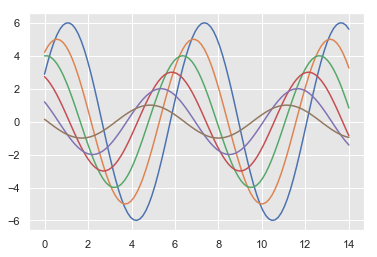seaborn11.png

### 绘图元素比例

``sns.set()``

``sns.set_context('paper')sinplot()``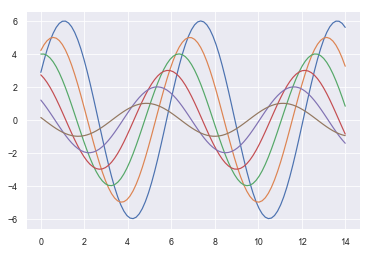seaborn12.png

``sns.set_context('talk')sinplot()``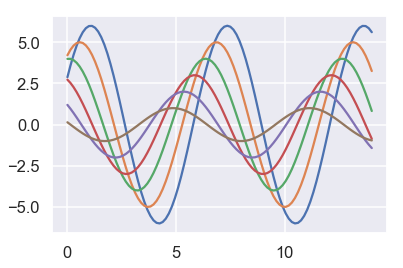seaborn13.png

``sns.set_context('poster')sinplot()``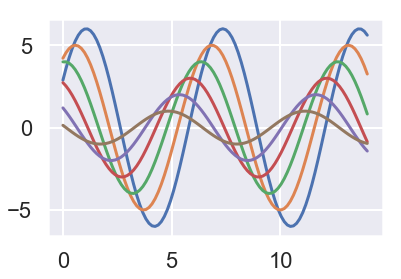seaborn14.png

``sns.set_context('notebook',font_scale = 1.5, rc = {'lines.linewidth':2.5})sinplot()``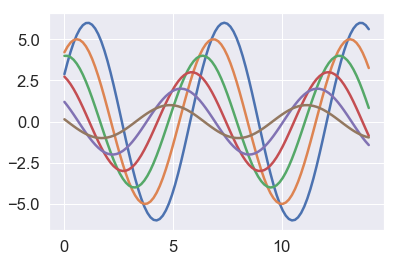seaborn15.png

阅读 100

阅读 101

阅读 137

阅读 116

阅读 136

阅读 562

阅读 1675

阅读 901

阅读 812

阅读 583